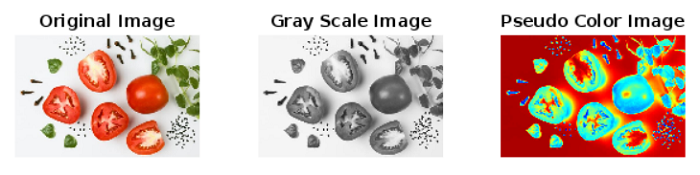# Gray Scale to Pseudo Color Transformation in MATLAB

MATLAB provides an easy and efficient way of processing images. In this tutorial, we will explore how to perform gray scale to pseudo color transformation in MATLAB.

In MATLAB, the gray scale to pseudo color transformation is an image processing technique which is used to create a visually appealing image by mapping the intensity values of the gray scale image to a specific color.

If we want to convert a gray scale image to a pseudo color image using MATLAB, then we can use a built-in function in MATLAB, which is ‘colormap’ and a color map matrix.

Now, let us discuss the step-by-step process to understand the process of gray scale to pseudo color transformation using MATLAB.

## Process of Converting Gray Scale Image to Pseudo Color Image

The step-by-step procedure to transform a gray scale image to pseudo color image is explained below:

Step (1) – Read the gray scale image.

Step (2) – Create a zero matrix of the same dimensions as the input gray scale image. Also, ensure that this matrix has three color channels for RGB (Red, Green, and Blue).

Step (3) – Create a colormap.

Step (4) – Extract the RGB color channels from the colormap matrix.

Step (5) – Calculate the color channel values for each pixel by using the intensity values in the grayscale image and store the resulting color information in the zero-matrix created in the second step.

Step (6) – Convert the color values stored in the zero matrix into a suitable format for image representation, for example 8-bit unsigned integer format.

Step (7) – Display the converted pseudo color image.

Hence, this is a straightforward algorithm in MATLAB programming which allows us to transform a gray scale image into a pseudo color image.

### Example

Now, let us consider an example in MATLAB to understand how to implement this algorithm in MATLAB programming to perform gray scale to pseudo color transformation.

% MATLAB program to perform gray scale to pseudo color transformation

% Convert input image to grayscale
gray_img = rgb2gray(img);

% Create an output image matrix (zero matrix)
out_img = zeros(size(gray_img, 1), size(gray_img, 2), 3);

% Specify a colormap
colormap_name = 'jet(256)';
color_map = colormap(colormap_name);

% Extract RGB color channels from the colormap matrix
red = color_map(:, 1);
green = color_map(:, 2);
blue = color_map(:, 3);

% Map intensity values of gray scale image to RGB color in the colormap
out_img(:, :, 1) = red(gray_img);
out_img(:, :, 2) = green(gray_img);
out_img(:, :, 3) = blue(gray_img);

% Convert the output image matrix to 8-bit unsigned integer format
out_img = im2uint8(out_img);

% Display the input image, grayscale image, and pseudo color transformed image
subplot(1, 3, 1); imshow(img); title('Original Image');
subplot(1, 3, 2); imshow(gray_img); title('Gray Scale Image');
subplot(1, 3, 3); imshow(out_img); title('Pseudo Color Image');


### Output### Explanation

This MATLAB code is written as per the steps explained in the above section. The only thing that I additionally included here is the input image is an RGB image which is first converted into a gray scale image and then performed its pseudo color transformation. You can see that output of the code for the image attached in the output sections. You can try this code with your own images.

## Conclusion

In conclusion, the gray scale to pseudo color transformation is an image processing technique which converts a gray scale image to its visually appealing representation. This transformation technique involves the use of colormap and a color map matrix to convert a gray scale image into a pseudo color image.

In this tutorial, we explained the concept and process of transforming a gray scale image into a pseudo color image with the help of an example program.

Updated on: 07-Sep-2023

54 Views